﻿ 基于双差定位方法的内蒙古中西部地区地震精确定位文章快速检索
 地震地磁观测与研究2021, Vol. 42Issue (1): 26-34  DOI: 10.3969/j.issn.1003-3246.2021.01.0050

### 引用本文GUO Wei, LIU Tiantian, FAN Lingling, et al. The accurate seismic location based on the double-difference method in the middle and western region of Inner Mongolia[J]. Seismological and Geomagnetic Observation and Research, 2021, 42(1): 26-34. DOI: 10.3969/j.issn.1003-3246.2021.01.005.### 文章历史

1. 中国内蒙古自治区 015331 乌加河地震台;
2. 中国北京 100036 中国地震局地震预测研究所;
3. 中国北京 100029 中国地震局地质研究所

The accurate seismic location based on the double-difference method in the middle and western region of Inner Mongolia
GUO Wei 1,2, LIU Tiantian 1, FAN Lingling 1, LI Guiming 1, ZHAO Ce 2, CHEN Hao 3
1. Wujiahe Seismic Station, Earthquake Agency of Inner Mongolia Autonomous Region, Wujiahe 015331, China;
2. Institute of Earthquake Forecasting, China Earthquake Administration, Beijing 100036, China;
3. Institute of Geology, China Earthquake Administration, Beijing 100029, China
Abstract: In view of the earthquake location deviation of the current seismic network, this paper uses the double-difference earthquake location method to relocate 4 410 earthquakes recorded by the Inner Mongolia seismic network and its surrounding stations from January 2008 to September 2019. The study shows that the earthquake distribution relocated by the double-difference method is more concentrated, more consistent with the geological structure characteristics of the western region of Inner Mongolia, significantly improving the location accuracy, and providing a more accurate research basis for improving the monitoring capability of Inner Mongolia seismic network.
Key words: double-difference earthquake location method    the central and western region of Inner Mongolia    accurate seismic location
0 引言

1 地震地质构造背景

2 资料选取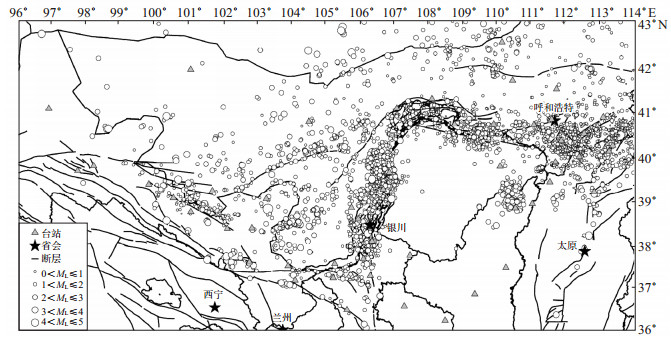图 1 研究区台站及地震震中分布 Fig.1 Distribution of seismic stations and earthquakes
3 原理方法

 $T_{k}^{i}=\tau^{i}+\int u \mathrm{~d} s$ (1)

 ${{x_i} = 111.199\left({{\lambda _i} - {\lambda _0}} \right)\cos \left({\frac{{{\phi _i} + {\phi _0}}}{2}} \right)}$ (2)
 ${{y_i} = 111.199\left({{\phi _i} - {\phi _0}} \right)}$ (3)

 $r_k^i = \sum\limits_{l = 1}^3 {\frac{{\partial T_k^i}}{{\partial x_l^i}}} \Delta x_l^i + \Delta {\tau ^i} + \int \delta u{\rm{d}}s$ (4)

 $r_k^j = \sum\limits_{l = 1}^3 {\frac{{\partial T_k^j}}{{\partial x_l^j}}} \Delta x_l^j + \Delta {\tau ^j} + \int \delta u{\rm{d}}s$ (5)

 $r_k^i - r_k^j = \sum\limits_{l = 1}^3 {\frac{{\partial T_k^i}}{{\partial x_l^i}}} \Delta x_l^i + \Delta {\tau ^i} + \int \delta u{\rm{d}}s - \sum\limits_{l = 1}^3 {\frac{{\partial T_k^j}}{{\partial x_l^j}}} \Delta x_l^j - \Delta {\tau ^j} - \int \delta u{\rm{d}}s$ (6)

 ${\rm{d}}r_k^{ij} = r_k^i - r_k^j = \sum\limits_{l = 1}^3 {\frac{{\partial T_k^i}}{{\partial x_l^i}}} \Delta x_l^i + \Delta {\tau ^i} - \sum\limits_{l = 1}^3 {\frac{{\partial T_k^j}}{{\partial x_l^j}}} \Delta x_l^j - \Delta {\tau ^j}$ (7)

 $\mathrm{d} r_{k}^{i j}=r_{k}^{i}-r_{k}^{j}=\left(T_{k}^{i}-T_{k}^{j}\right)^{\mathrm{obs}}-\left(T_{k}^{i}-T_{k}^{j}\right)^{\mathrm{cal}}$ (8)

4 双差定位结果及分析 4.1 参数选取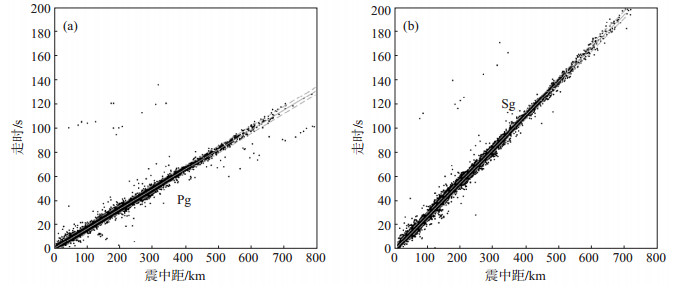图 2 P波和S波走时—震中距曲线 （a）P波；（b）S波；中间直线是拟合的平均时距曲线；两侧直线分别是±5 s的时距曲线 Fig.2 Travel time-distance curves of P(left column)and S(right column) waves表 1 内蒙古中西部地区水平层状速度模型 Table 1 Layered velocity model for the central and western regions of Inner Mongolia
4.2 结果分析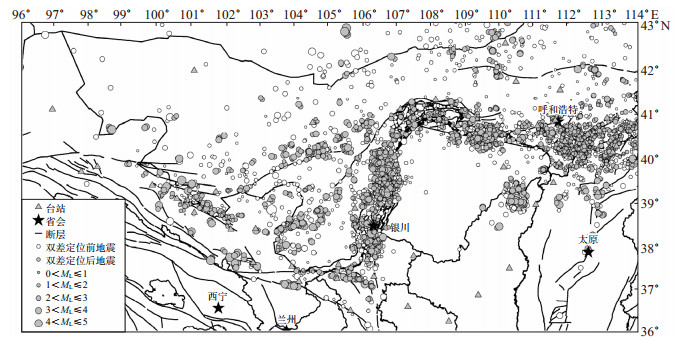图 3 重定位前后地震震中分布 Fig.3 Epicenter distributions before and after double-difference relocation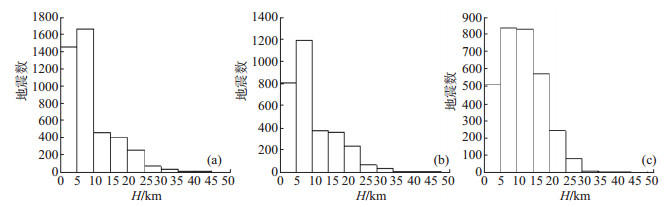图 4 重定位前后震源深度分布 （a）4 410个地震事件的震源深度分布；（b）双差定位后得到的3 100个地震事件重定位前的震源深度分布；（c）重定位后3 100个地震事件的震源深度分布 Fig.4 Statistics of focal depths before and after relocation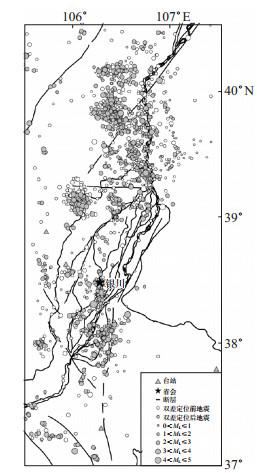图 5 黄河流域附近地区 地震重定位结果 Fig.5 Double-difference relocation near the Yellow River basin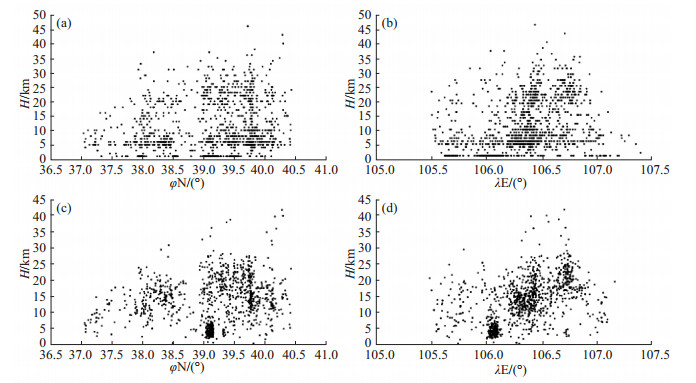图 6 重定位前后震源深度随经纬度的分布 （a）、（b）重定位前；（c）、（d）重定位后 Fig.6 Distribution of focal depth with latitude and longitude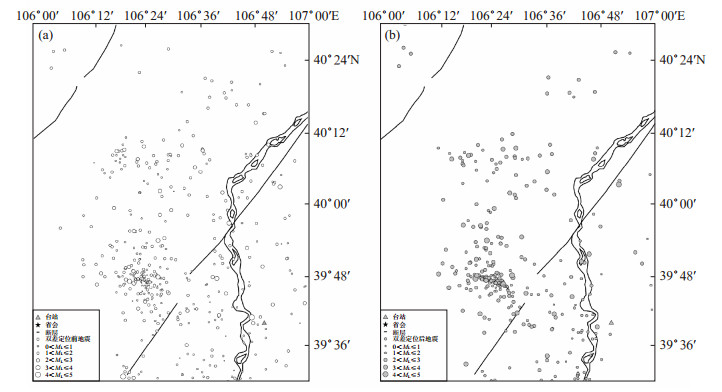图 7 乌海台附近地区重定位前后地震震中分布 （a）重定位前；（b）重定位后 Fig.7 Distribution map of epicenters before and after relocation near Wuhai Seismic Station
5 结论与讨论

(1) 双差定位后精度更高，均方根残差平方和RMS较小。通过双差定位得到的三分向误差均为1 km左右，均方根残差平方和RMS为0.18 s。

(2) 双差定位后地震震中分布更集中，呈现较明确的沿断裂带密集分布的特征，鄂尔多斯块体西北缘附近地震分布特征尤为明显，揭示了研究区内地震活动与活动构造间的密切关系。

(3) 重定位后震源深度集中分布在10—20 km，重定位前震源深度分布在10 km以内，重定位后震源较定位前更深，基本上都集中分布在上部地壳之中，这可能揭示了内蒙古中西部地区地壳相对较深的特征。

 白玲, 张天中, 张宏智. 多重相对定位法和波形相关校正及其应用[J]. 地震学报, 2003, 25(6): 591-600. DOI:10.3321/j.issn:0253-3782.2003.06.004 陈凯, 孙国栋, 易江, 等. 重庆及邻区地震精定位及活动性分析[J]. 地震地磁观测与研究, 2019, 40(5): 8-20. 黄媛, 杨建思, 张天中. 2003年新疆巴楚-伽师地震序列的双差法重新定位研究[J]. 地球物理学报, 2006, 49(1): 162-169. DOI:10.3321/j.issn:0001-5733.2006.01.022 李彬, 魏建民, 张浩鸣, 等. 2015年内蒙古阿拉善左旗5.8级地震序列震源深度对比分析[J]. 地震地磁观测与研究, 2018, 39(4): 38-46. DOI:10.3969/j.issn.1003-3246.2018.04.006 刘芳, 宋秀青, 张帆, 等. 内蒙古地区地壳速度模型研究[J]. 地震地磁观测与研究, 2016, 37(2): 26-34. 刘芳, 宋秀青, 张帆, 等. 内蒙古地区地壳速度模型在地震定位中的应用[J]. 地震地磁观测与研究, 2016, 37(4): 27-32. 刘希强, 周彦文, 曲均浩, 等. 应用单台垂向记录进行区域地震事件实时检测和直达P波初动自动识别[J]. 地震学报, 2009, 31(3): 260-271. DOI:10.3321/j.issn:0253-3782.2009.03.003 柳云龙. 西南印度洋洋中脊龙旂热液区地震活动及其构造特征研究[D]. 长春: 吉林大学, 2018. 罗佳宏. 长江三峡库区三维速度成像与微震活动性研究[D]. 北京: 中国地震局地质研究所, 2016. 钱佳威. 矿山微地震的四维成像[C]//2015中国地球科学联合学术年会论文集(二十三)——专题55煤炭资源与矿山安全地球物理. 北京: 中国地球物理学会, 全国岩石学与地球动力学研讨会组委会, 中国地质学会构造地质学与地球动力学专业委员会, 中国地质学会区域地质与成矿专业委员会, 2015: 4. 王伟平. 鲜水河、安宁河和龙门山断裂带交汇区双差层析成像研究[D]. 北京: 中国地震局地球物理研究所, 2016. 肖卓, 高原. 利用双差成像方法反演青藏高原东北缘及其邻区地壳速度结构[J]. 地球物理学报, 2017, 60(6): 2213-2225. 尹战军, 张帆, 郝美仙, 等. 利用双差定位方法研究地震震源深度[J]. 山西地震, 2018, 11061106(1): 4-6. DOI:10.3969/j.issn.1000-6265.2018.01.002 张帆, 刘芳, 张晖. 岱海断陷带地区地震重新定位研究[J]. 地震地磁观测与研究, 2014, 35(1/2): 69-74. 张帆, 刘芳, 张晖, 等. 内蒙古中西部一维均匀地壳速度模型研究[J]. 地震地磁观测与研究, 2015, 36(4): 1-5. 张帆, 刘永梅, 郝美仙, 等. 内蒙古阿拉善地区几次中等强度地震震源深度测定[J]. 地震地磁观测与研究, 2017, 38(2): 38-45. DOI:10.3969/j.issn.1003-3246.2017.02.008 张国民, 汪素云, 李丽, 等. 中国大陆地震震源深度及其构造含义[J]. 科学通报, 2002, 47(9): 663-668. DOI:10.3321/j.issn:0023-074X.2002.09.004 张娜, 赵翠萍, 周连庆. 三峡水库区上地壳三维精细速度结构成像[J]. 地震, 2018, 38(4): 37-48. DOI:10.3969/j.issn.1000-3274.2018.04.004 张天中, 武巴特尔, 黄媛, 等. 近台资料对近震相对定位算法的影响[J]. 地球物理学报, 2007, 50(4): 1123-1130. DOI:10.3321/j.issn:0001-5733.2007.04.019 赵翠萍. 1997-2003年新疆伽师震源区特征的地震学方法研究[D]. 北京: 中国地震局地球物理研究所, 2006. 赵艳红, 舒雷, 刘永梅, 等. 内蒙古分区地壳速度模型的建立及应用分析[J]. 中国地震, 2018, 34(4): 731-744. DOI:10.3969/j.issn.1001-4683.2018.04.013 赵杨. 地震体波走时与重力联合反演研究及在南北地震带南段的应用[D]. 北京: 中国地质大学(北京), 2018. 朱艾斓, 徐锡伟, 胡平, 等. 首都圈地区小震重新定位及其在地震构造研究中的应用[J]. 地质论评, 2005, 51(3): 268-274. DOI:10.3321/j.issn:0371-5736.2005.03.007 左可桢. 门源地区震源位置及三维速度结构研究[D]. 兰州: 中国地震局兰州地震研究所, 2018. Waldhauser F, Ellsworth W L. A double-difference earthquake location algorithm: method and application to the Northern Hayward Fault, California[J]. Bull Seismol Soc Am, 2000, 90(6): 1353-1368. DOI:10.1785/0120000006